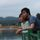cancel
Showing results for
Did you mean:Helper IV

## Variance vs. Budget and Filters

I have a table of projects, expenses and budgets.  I wanted to calculated variance from budget then do some filtering on those that are say 10,000 under budget. I have run into a few problems

I used a new column of Variance = table1[actuals ytd] - table1[budget ytd]

However if there are multiple lines for a project I get the variance per line when I need it aggregated at the project level.

I get:

Proj1  Amount1  variance 1

Proj1 Amount2  variance 2

Proj1 Amount3  variance 3

Proj2 Amount4 variance 4

Proj2 Amount5  variance 5

Proj2 Amount6  variance 6

Proj2 Amount7  variance 7

I really just need Project 1 and Project 2 the variances aggregated.

Seems like it should be simple, but looks to be hard.

then after i would want to be able to filter on variance totals.

Any thoughts?

Alan

1 ACCEPTED SOLUTIONMicrosoft

No that should work.  If you put a chart/table in your report with Project Number as axis and the measure as the value, it will calculate that measure for each Project Number as if that were a filter applied to the underlying data.

In more complex examples, there may be performance differences between calculated DAX columns and columns created in the query editor.  The query editor generally will apply query folding and push the processing workload to the data source (where applicable), whereas the calculated DAX column happens within Power BI.  DAX is way more flexible than the query editor, however, so my best advice is to do the ETL and mashing phase as much as possible in the query editor, but save the advanced pivoting and calculating for DAX.

5 REPLIES 5Microsoft

If you just want a measure, you could use something like the following:

Proj1Variance = Calculate(Sum(TableName[Variance]),Filter(TableName, [Project] = "Proj1"))Microsoft

I would create the calculated variance column in the query editor, then use the "Group By" function to group rows by Project and sum the variance column.Helper IV

I also just changed the calculated column to a measure and it seemed to work.

YTD Variance = sum(CurYear[Actuals YTD]) - sum( CurYear[Budget YTD])

am i missing something as to why this might be bad?

thanks again

AlanMemorable Member

Hi @asjones,

The formula of Calculated Column and Calculated Measure in your scenario are same.

Did you select "Don't summarize" for actual & budget? it could be the reason to force data in table showing row by rowMicrosoft

No that should work.  If you put a chart/table in your report with Project Number as axis and the measure as the value, it will calculate that measure for each Project Number as if that were a filter applied to the underlying data.

In more complex examples, there may be performance differences between calculated DAX columns and columns created in the query editor.  The query editor generally will apply query folding and push the processing workload to the data source (where applicable), whereas the calculated DAX column happens within Power BI.  DAX is way more flexible than the query editor, however, so my best advice is to do the ETL and mashing phase as much as possible in the query editor, but save the advanced pivoting and calculating for DAX.Announcements#### Exclusive opportunity for Women!

Join us for a free, hands-on Microsoft workshop led by women trainers for women where you will learn how to build a Dashboard in a Day!#### Power Platform Conference-Power BI and Fabric Sessions

Join us Oct 1 - 6 in Las Vegas for the Microsoft Power Platform Conference.Top Solution Authors
Top Kudoed Authors
Users online (2,170)